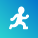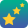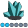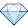## New macro functions %EvalC and %EvalN

Because the macro language is not typed (i.e. all values are text strings, rather than being defined as numeric or character) both %Eval and %SysEvalF must do some "guessing" to determine whether to treat text string as a character value or a numeric value.  I would propose to add %EvalC which would always to character evaluation (even if the argument looked like a number) and %EvalN which would always do numeric evaluation (and if the argument could not be interpreted as a number, would return an error).

For example,

1.

`%put >>%eval(5.0>15)<<;`

Returns true, because %eval does not know about decimals.  When it sees the decimal, it decides the argument is a character string and does a character (alphabetical) comparison.  This is probably not then result intended by the person who wrote the SAS code.

2.

``%put >>%sysevalf(5.0>15)<<;``

Returns false.  %SYSEVALF knows that decimals are numbers and therefore does a numeric comparison as intended.  %SYEVALF also knows about SAS date literalals, and lots of other numbers, which is good.

3.

``%put >>%sysevalf(793221D360=)<<;``

Fails (on linux 9.3) with an overflow error.  Here, the user intended a character comparison to check for null, which should return false.  But %SYSVALF saw a number written in exponential notation (D is interpretted same as E) which caused the overflow.

I think these examples all result from the flexibility designed into %EVAL and %SYSEVALF to handle both character values and numeric values.  I would prefer an additional pair of functions which did NOT have this flexibity.   So %EvalN(5.0>15) would return true, because it would know that decimals are numeric (and dates and exponential notation and everything else %sysevalf knows).  But %EvalN(b>a) would return an ERROR, because the values could not be intrepreted as numeric.  Similarly %EvalN(1e2=100) would return true, but %EvalC(1e2=100) would return false, because %EvalC would do a character comparison despite the fact that the argument looks like a number.

Without these functions, if I want to force %EVAL to do a character comparison, a hack workaround is to add a text character so that it cannot look like a number, e.g. %EVAL(Q&a=Q&b).  But that's ugly.  And I don't think there's a good way to force %EVAL to do a numeric comparison or error on a character value  (without inserting my own logic to catch character values).

As a developer, if I write a %IF statement (with implied %eval) or explicit %EVAL / %SYSEVALF, in my design mind I know whether I am intending a numeric comparison or a character comparison.  It would be useful to be able to communicate this intent in my code, rather than let %EVAL / %SYSEVALF guess as to my intent.SAS Employee

What about adding a character 'conversion-type' to %sysevalf to signify to treat the input as character data?  I think it would also be simpler to keep %eval as is, optionally just adding %evalC, even though it wouldn't benefit example #1PROC Star

Thanks @FriedEgg .  I agree with leaving %Eval as is.

My goal is to be able to specify that a boolean expression should be evaluated as a character expression vs a numeric expression.  I like %EvalC and %EvalN for that, partly because %SysEvalF() always felt like a long name for what I wish %EVAL did.  But I think I could also agree with a %SysEvalF approach, if it allowed me to specify both character and numeric evaluation.  Currently the Boolean conversion-type does both character evaluations and numeric evaluations, without allowing me to specify my intent.  Maybe adding a BooleanC type and a BooleanN type would work for me.  So:

``````%put >>%sysevalf(5.0>15,BooleanN)<<; *False- numeric comparison;
%put >>%sysevalf(5.0>15,BooleanC)<<; *True- character comparison;
%put >>%sysevalf(5.0>Q15,BooleanN)<<; *Error: character operand found where numeric expected;

``````Fluorite | Level 6

I recommend allowing strong numeric and character typing of the results of an evaluation of an argument. If one knows the expected data type of the result, the more specific evaluation will prevent potentially erroneous choices by the compiler.

_S_Diamond | Level 26

I recommmend using Base SAS which does have constructs for each datatype, and has functions to process these, and is in its entirety what it should be used for.  Macro language is only present to generate Base SAS code.  It does nothing else, and I really don't understand this need to push Base SAS out of the picture and only use Macro language?  Its a bit like saying, sure the enhanced editor (in an ideal world) has code recognition, finds functions, runs things, shows log etc. but I have to use Word to code my programs.Fluorite | Level 6

The SAS Macro language has evolved as a way to operate on the computing environment of SAS/Base. For example, one may need to concatenate the values of %SysVer() and the OS to determine which compiled Macro library and Format library to reference. A relatively simple SAS Macro places the label of the global environment prior to SAS/Base program compilation.

_S_PROC Star

Agreed, @RW9, that the primary purpose of the macro language is to generate SAS code, and the DATA step language is designed to manipulate data.  Still, there are many places where even basic use of the macro language requires it to evaluate a logical expression, and it implicitly calls %EVAL.  This is done in %IF statements, %DO loops, etc.  This is the situation where I would like to be able to specify that an expression should be evaluated as a numeric expression vs character expression.

I'm not trying to replicate the power of the DATA step in manipulating data, but nor do I want to have to drop out of the macro language and into the DATA step language every time I want to evaluate the value of some macro variable (in order make some decision about what SAS code to generate).

As an example, when given two macro vars X and Y, I think it's good that we can code:

``%if &x < &y %then %do ... ;``

``````data _null_;
call symputx("boolean",&x < &y);
run;
%if &boolean %then %do ... ;``````

A macro language without  %IF statements or %DO loops would not be very useful.

I just want to have a way to speficy in the macro language whether the expression &x<&y should be evaulated as a numeric comparison or a character expression.Super User

If the actual issue is that your "users" can't provide clean data for values (your 793221D360 example) then before any processing the rules for the type of intended variable should be enforced. You could check the contents using %sysfunc and the various ANYALPHA ANYCNTRL ANYGRAPH ANYPUNCT or similar functions before passing to the %eval or %sysevalf functions (or places that use them implicitly such as %if)PROC Star

Thanks @ballardw.  It isn't always a problem of users entering bad values. I think it's more that as a developer, I want to state which type of evaluation (numeric or character) should be done.  For example, a user may be allowed to pass &x=10 &y=2.  I should then be able to dictate whether &x > &y does a character comparison (return false) or numeric comparison (return true).  I think the flexibility designed into %Eval and %SysEvalF to do both character and numeric evaluation is problematic.

Yes, I could use %ANYALPHA, or the SAS-provided %DataTyp autocall macro (copied below) to try to determine type.  But to me, that introduces another layer of guessing.  That is, I look at a text value and guess through some algorithm whether it is a number or a character string and make some conditional decision, and then call %SysEvalF which will also look at the text value and also guess whether it is a number or a character using (likely) some different algorithm and make a conditional decision.  So I still risk my algorithm guessing a string is numeric, and sending that value to %SysEvalF only to have %SysEvalF suprise me and treat it as character (without an indication in the log).

My goal in recommending %EvalC and %EvalN is to avoid such guessing.

``````%macro datatyp(parm);
%*********************************************************************;
%*                                                                   *;
%*  MACRO: DATATYP                                                   *;
%*                                                                   *;
%*  USAGE: %datatyp(parm)                                            *;
%*                                                                   *;
%*  DESCRIPTION:                                                     *;
%*    The DATATYP macro determines if the input parameter is         *;
%*    NUMERIC or CHARacter data, and returns either CHAR or NUMERIC  *;
%*    depending on the value passed in through parm.                 *;
%*                                                                   *;
%*  PROCEDURE:                                                       *;
%*    This macro checks first removes leading and trailing blanks.   *;
%*    Then it checks for and removes a sign character (+ or -).      *;
%*    Then it checks for a string of digits, a decimal point, or     *;
%*    a floating point exponent, followed by an optional sign and    *;
%*    a string of digits.                                            *;
%*                                                                   *;
%*  ERRORS/RESTRICTIONS:                                             *;
%*    This macro requires the %VERIFY macro.  Commas are not         *;
%*    allowed as the SAS word scanner does not accept them.  It      *;
%*    is not guaranteed that a string judged NUMERIC will in fact    *;
%*    be acceptable to the SAS word scanner as no range checking     *;
%*    is done.                                                       *;
%*                                                                   *;
%*********************************************************************;
%local type char len pos fract expon;
%let type=CHAR;
%let parm=%qleft(%qtrim(&parm));
%let len = %length(&parm);
%if &len > 0 %then %do;
%if %verify(&parm,%str(0123456789+-.EeDd))=0 %then %do;
%let char = %qsubstr(&parm,1,1);
%if &char=%str(+) | &char=%str(-) %then %do;
%if &len < 2 %then %let parm = ;
%else %let parm = %qsubstr(&parm,2);
%let len = %eval(&len - 1);
%end;
%let fract = 0;
%let expon = 0;
%repeat: %if &len > 0 %then %do;
%let pos = %verify(&parm,0123456789);
%if &pos = 0 %then %let type=NUMERIC;
%else %do;
%if &len > &pos %then %let parm = %qsubstr(&parm,&pos);
%let len = %length(&parm);
%let char = %qsubstr(&parm,1,1);
%if &char=%str(.) & &fract=0 & &expon=0 %then %do;
%if &len < 2 %then %let parm = ;
%else %let parm = %qsubstr(&parm,2);
%let len = %eval(&len - 1);
%let fract = 1;
%goto repeat;
%end;
%else %if (&char=%str(E) | &char=%str(e) |
&char=%str(D) | &char=%str(d)) &
&expon=0 & &pos>1 & &len>1 %then %do;
%let parm = %qsubstr(&parm,2);
%let len = %eval(&len - 1);
%let char = %qsubstr(&parm,1,1);
%if &char=%str(+) | &char=%str(-) %then %do;
%if &len < 2 %then %let parm = ;
%else %let parm = %qsubstr(&parm,2);
%let len = %eval(&len - 1);
%end;
%let expon = 1;
%goto repeat;
%end;
%end;
%end;
%end;
%end;
&type
%mend datatyp;``````Tourmaline | Level 20

> Currently the Boolean conversion-type does both character evaluations and numeric evaluations, without allowing me to specify my intent.

Late to the game, but the guesswork can easily be avoided by quoting the tested values to force string comparisons

This makes the intent obvious.

``````%let a=5.1;
%let b=15e4;
%put 1-%sysevalf( &a > &b )/%sysevalf( &a < &b );
%put 2-%sysevalf("&a">"&b")/%sysevalf("&a"<"&b");
``````

1-0/1
2-1/0Tourmaline | Level 20

This behaviour can even be managed though a resettable parameter.

``````%let a=5.1;
%let b=15e4;
%let q=;
%put 1-%sysevalf(&q&a&q>&q&b&q)/%sysevalf(&q&a&q<&q&b&q);
%let q=%str(%");
%put 2-%sysevalf(&q&a&q>&q&b&q)/%sysevalf(&q&a&q<&q&b&q);
``````

1-0/1
2-1/0